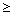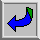## Zeno: Argument against Plurality

1. #### Introduction

The argument is contained in 4=B1 and 3=B2 (from Simplicius’ commentary on Aristotle’s Physics). But there is a problem with the text, and some of the argument is garbled or lost. Fortunately, we can reconstruct it. Zeno attempts to show that the assumption that there are many things leads to a contradiction: viz., that each thing is both infinitely small and infinitely large.

There are two limbs to the argument. The pluralist’s assumption, “There are many things,” leads to these two conclusions:

1. Each thing is “so small as not to have size.”
2. Each thing is “so large as to be unlimited.”

Simplicius’s text does not preserve (A) completely. It starts with (A), and then is garbled and switches over to (B). But we can reconstruct the argument for (B).

2. #### The Argument

Simplicius (in 4=B1) preserves one key principle (“if it exists, each thing must have some size and thickness”). It is a premise that Zeno thinks his materialist/pluralist opponents must accept.  3=B2 contains an argument in support of this principle (“Suppose that x has no size. Then when x is added to a thing it does not increase the size of that thing, and when x is subtracted from a thing, that thing does not decrease in size. Clearly, x is nothing, i.e., does not exist.”). So the argument begins with this premise:

1. What exists has size (magnitude).

Zeno also seems to be making the following two assumtions:

2. What has size can be divided into (proper) parts that exist.

3. The part of relation is transitive, irreflexive, and asymmetical.

 Proper parts: x is a proper part of y iff x is a part of y and y is not a part of x Transitive: if x is a part of y and y is a part of z, then x is a part of z. Irreflexive: x is not a part of x. Asymmetical: if x is a part of y, then y is not a part of x.

The rest of his argument is preserved in 4=B1. Roughly paraphrased, it runs:

4. Pick any existing physical object, x.

5. x has size. [from 1 and 4]

6. x has parts. [from 2 and 5]

7. Let x' be one of those parts; then x' “must be apart from the rest” of x. That is, one part of x must protrude, or “be in front” of the rest of x, as Zeno goes on to say.

Now Zeno says that the same argument applies to x'!

8. So some part of x' (call it x'') protrudes from the rest of x', and so on, ad infinitum.

Since Zeno is assuming, reasonably enough, that the part of relation is transitive (i.e., that the parts of the parts of x are also parts of x) it follows that x is composed of an infinite number of parts (since x', x'', x''', etc., ad infinitum, are all parts of x).

9. So x has infinitely many parts. [from 8 and 3]

Zeno immediately infers that such an object (with infinitely many parts) must be infinitely large.

10. So x is infinitely large. [from 9 and ?]

3. #### Evaluation of the argument

Everything is fine up to step (9). But (9) does not entail (10). Zeno seems to be implicitly assuming (what I’ll call) the Infinite Sum Principle: viz., that the sum of an infinite number of terms is infinitely large. (9), together with the Infinite Sum Principle, entails (10). And from (10) it follows (by Universal Generalization) that every magnitude is infinitely large, which is the conclusion of the second limb.

The Infinite Sum Principle appears to be correct. But is it? What makes it seem correct is the observation that you can make something as large (a finite size) as you want out of parts as small as you want, and it takes only a finite number of them to do this! To see that this is so, consider the following: pick any magnitude, y, as large as you like; and pick any small magnitude, z, as small as you like (but z > 0). It is obvious that you can obtain a magnitude at least as large as y by adding z to itself a finite number of times. That is:

yzx (x · zy)

For every y and for every z, there is at least one x such that x times z is greater than or equal to y.

If y and z are finite, no matter how large y is and no matter how small z is, if you have enough things (but still only finitely many) each of which is at least as large as z you get a total magnitude at least as large as y. So, the reasoning goes, if you had an infinite number of z’s, you’d get an infinitely large sum.

This may seem convincing, but it doesn’t support the Infinite Sum Principle. For this argument has been assuming that of our infinitely many parts, there is a smallest. (More precisely, there is one than which none is smaller.) What our argument actually supports is only an amended version of the Infinite Sum Principle.

The sum of an infinite number of terms, one of which is the smallest, is infinite.

This amended principle is true. But it won’t help Zeno. For in his series there is no smallest term! That is, x' is smaller than x, and x'' is smaller than x', etc. We have an infinite series of continually decreasing terms. And the sum of such a series may be finite.

E.g.: 1/2 + 1/4 + 1/8 + . . .+ . . .= 1.

[If this last point seems puzzling, you need to learn a little about infinite sequences, limits of infinite sequences, infinite series, and sums of infinite series.  Please take a moment to study the mathematical background to Zeno’s paradoxes.]

4. #### Review

Zeno’s argument is based on two principles:

• Infinite Divisibility Principle

• Infinite Sum Principle

He gives a compelling argument for the first, but does not even mention the second. From these he infers his conclusion that every magnitude is infinitely large.

This argument is valid, but unsound. For the Infinite Sum Principle is false.

We can fix the Infinite Sum Principle by restricting it to infinite sets with smallest elements. The amended principle is true, and so the resulting argument’s premises are both true. But this amended argument is invalid. For the amended principle requires that there be smallest parts, and the Infinite Divisibility Principle does not guarantee that there are such parts - it allows the parts to get smaller and smaller, ad infinitum.

We can make Zeno’s argument valid, but then one of its premises is false. Or we can make both of its premises true, but then it is invalid. Either way, Zeno’s argument is unsound.Go to next lecture on Zeno’s race course, part 2Go to previous lecture on Zeno’s race course, part 1Return to the PHIL 320 Home Page